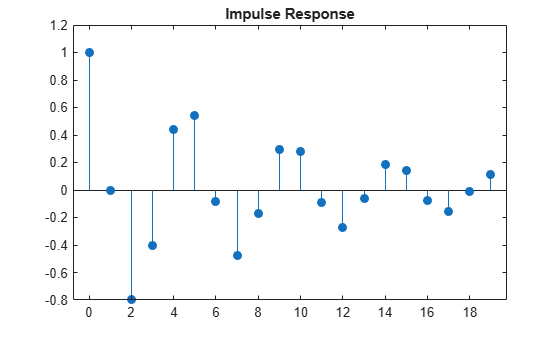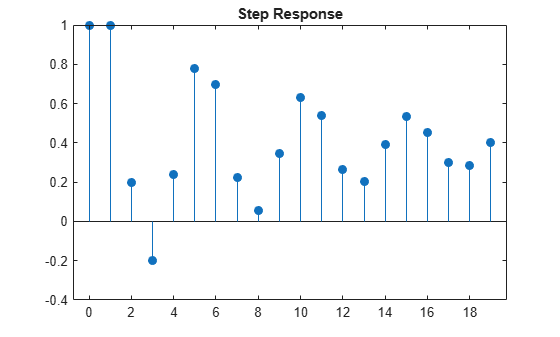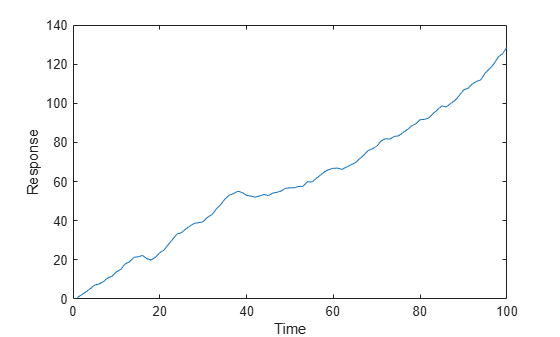Documentation

# filter

Filter disturbances using ARIMA or ARIMAX model

## Syntax

```[Y,E,V] = filter(Mdl,Z) [Y,E,V] = filter(Mdl,Z,Name,Value) ```

## Description

```[Y,E,V] = filter(Mdl,Z)``` filters disturbances, `Z`, to produce responses, innovations, and conditional variances of a univariate ARIMA(p,D,q) model.

```[Y,E,V] = filter(Mdl,Z,Name,Value)``` filters disturbances using additional options specified by one or more `Name,Value` pair arguments.

## Input Arguments

`Mdl`

ARIMA model, as created by `arima` or `estimate`. The input model cannot have any `NaN` values.

`Z`

`numObs`-by-`NumPaths` matrix of disturbances, zt, that drives the innovation process, εt. For a variance process ${\sigma }_{t}^{2},$ the innovation process is given by

`${\epsilon }_{t}={\sigma }_{t}{z}_{t}.$`

As a column vector, `Z` represents a path of the underlying disturbance series. As a matrix, `Z` represents `numObs` observations of `NumPaths` paths of the underlying disturbance series. `filter` assumes that observations across any row occur simultaneously. The last row contains the most recent observation.

### Note

`NaN`s indicate missing values. `filter` removes them from `Z` using list-wise deletion. That is, `filter` removes any row of `Z` containing at least one `NaN`. This deletion reduces the effective sample size and can cause irregular time series.

### Name-Value Pair Arguments

Specify optional comma-separated pairs of `Name,Value` arguments. `Name` is the argument name and `Value` is the corresponding value. `Name` must appear inside quotes. You can specify several name and value pair arguments in any order as `Name1,Value1,...,NameN,ValueN`.

 `'V0'` Positive presample conditional variances that provide initial values for the model. If `V0` is a column vector, then `filter` applies it to each output path. If `V0` is a matrix, then it requires at least `NumPaths` columns. If the number of columns exceeds `NumPaths`, then `filter` uses the first `NumPaths` columns. `V0` requires enough rows to initialize the moving average component of the ARIMA model and any conditional variance model. The required number of rows is at least `Mdl.Q`. If you include a conditional variance model, then `filter` might require more than `Mdl.Q` rows. If the number of rows in `V0` exceeds the number necessary, then `filter` uses the most recent observations. The last row contains the most recent observation. Default: `filter` sets necessary presample observations to the unconditional variance of the conditional variance process. `'X'` Matrix of predictor data corresponding to a regression component in the conditional mean model. The columns of `X` are separate, synchronized time series, with the last row containing the most recent observations. The number of rows of `X` must be at least the number of rows of `Z`. When the number of rows of `X` exceeds the number necessary, then `filter` uses the most recent observations. Default: `filter` does not include a regression component in the conditional mean model regardless of the presence of regression coefficients in `Mdl`. `'Y0'` Presample response data, providing initial values for the model. If `Y0` is a column vector, then `filter` applies it to each output path. If `Y0` is a matrix, then it requires at least `NumPaths` columns. If the number of columns in `Y0` exceeds `NumPaths`, then `filter` uses the first `NumPaths` columns. `Y0` requires at least `Mdl.P` rows to initialize the model. If the number of rows in `Y0` exceeds `Mdl.P`, then `filter` uses the most recent `Mdl.P` observations. The last row contains the most recent observation. Default: `filter` sets the necessary presample observations to the unconditional mean for stationary processes, and to 0 for nonstationary processes or processes with a regression component. `'Z0'` Presample disturbances, providing initial values for the input disturbance series, `Z`. If `Z0` is a column vector, then `filter` applies it to each output path. If `Z0` is a matrix, then it requires at least `NumPaths` columns. If the number of columns exceeds `NumPaths`, then `filter` uses the first `NumPaths` columns. `Z0` requires a sufficient number of rows to initialize the moving average component of the ARIMA model and any conditional variance model. The required number of rows is at least `Mdl.Q`, but might be more if a conditional variance model is included. If the number of rows in `Z0` exceeds the number necessary, then `filter` uses the most recent observations. The last row contains the most recent observation. Default: `filter` sets the necessary presample observations to 0.

### Notes

• `NaN`s in the data indicate missing values and `filter` removes them. The software merges the presample data and main data sets separately, then uses list-wise deletion to remove any `NaN`s. That is, `filter` sets `PreSample` = ```[Y0 Z0 V0]``` and `Data` = `[Z X]`, then it removes any row in `PreSample` or `Data` that contains at least one `NaN`.

• Removing `NaN`s in the main data reduces the effective sample size. Such removal can also create irregular time series.

• `filter` assumes that you synchronize presample data such that the most recent observation of each presample series occurs simultaneously.

• All predictor series in `X` (i.e., columns of `X`) are applied to each disturbance series in `Z` to produce `NumPaths` response series `Y`.

## Output Arguments

 `Y` `numObs`-by-`NumPaths` matrix of simulated responses. `Y` is the continuation of the presample series `Y0`. `E` `numObs`-by-`NumPaths` matrix of simulated innovations with conditional variances, `V`. Each column is a scaled series of innovations (or disturbances) such that `E = sqrt(V)*Z`. `V` `numObs`-by-`NumPaths` matrix of conditional variances of the innovations in `E` such that `E = sqrt(V)*Z`. `V` is the continuation of the presample series `V0`.

## Examples

expand all

Specify a mean zero ARIMA(2,0,1) model.

```Mdl = arima('Constant',0,'AR',{0.5,-0.8},'MA',-0.5,... 'Variance',0.1);```

Simulate the model using Monte Carlo simulation. Then, standardize the simulated innovations and filter them.

```rng(1); % For reproducibility [y,e,v] = simulate(Mdl,100); Z = e./sqrt(v); [Y,E,V] = filter(Mdl,Z);```

Confirm that the outputs of `simulate` and `filter` are identical.

`isequal(y,Y)`
```ans = logical 1 ```

The logical value `1` confirms the two outputs are identical.

Specify a mean zero ARIMA(2,0,1) model.

```Mdl = arima('Constant',0,'AR',{0.5,-0.8},'MA',-0.5,... 'Variance',0.1);```

Simulate the first 20 responses of the impulse response function. Generate a disturbance series with a one-time, unit impulse, and then filter it. Set all presample observations equal to zero. Normalize the impulse response function to ensure that the first element is 1.

```Z = [1;zeros(19,1)]; Y = filter(Mdl,Z,'Y0',zeros(Mdl.P,1)); Y = Y/Y(1);```

Plot the impulse response function.

```figure; stem((0:numel(Y)-1)',Y,'filled'); title 'Impulse Response';```The impulse response assesses the dynamic behavior of a system to a one-time, unit impulse. You can also use the `impulse` method to plot the impulse response function for an ARIMA process.

Assess the dynamic behavior of a system to a persistent change in a variable by plotting a step response.

Specify a mean zero ARIMA(2,0,1) process.

```Mdl = arima('Constant',0,'AR',{0.5,-0.8},'MA',-0.5,... 'Variance',0.1);```

Simulate the first 20 responses to a sequence of unit disturbances. Generate a disturbance series of ones, and then filter it. Set all presample observations equal to zero.

```Z = ones(20,1); Y = filter(Mdl,Z,'Y0',zeros(Mdl.P,1)); Y = Y/Y(1);```

The last step normalizes the step response function to ensure that the first element is 1.

Plot the step response function.

```figure; stem((0:numel(Y)-1)',Y,'filled'); title 'Step Response';```Create models for the response and predictor series. Set an ARIMAX(2,1,3) model to the response `MdlY`, and an AR(1) model to the `MdlX`.

```MdlY = arima('AR',{0.1 0.2},'D',1,'MA',{-0.1 0.1 0.05},... 'Constant',1,'Variance',0.5, 'Beta',2); MdlX = arima('AR',0.5,'Constant',0,'Variance',0.1);```

Simulate a length 100 predictor series `x` and a series of iid normal disturbances `z` having mean zero and variance 1.

```rng(1); z = randn(100,1); x = simulate(MdlX,100);```

Filter the disturbances `z` using `MdlY` to produce the response series `y`, and plot `y`.

```y = filter(MdlY,z,'X',x); figure; plot(y); title 'Filter to simulate ARIMA(2,1,3)'; xlabel 'Time'; ylabel 'Response';```## Alternatives

• `filter` generalizes `simulate`. That is, both filter a series of disturbances to produce output responses, innovations, and conditional variances. However, `simulate` autogenerates a series of mean zero, unit variance, independent and identically distributed (iid) disturbances according to the distribution in `Mdl`. In contrast, `filter` lets you directly specify your own disturbances.

## References

 Box, G. E. P., G. M. Jenkins, and G. C. Reinsel. Time Series Analysis: Forecasting and Control 3rd ed. Englewood Cliffs, NJ: Prentice Hall, 1994.

 Enders, W. Applied Econometric Time Series. Hoboken, NJ: John Wiley & Sons, 1995.

 Hamilton, J. D. Time Series Analysis. Princeton, NJ: Princeton University Press, 1994.